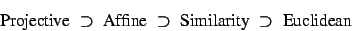Next: From Projective to Affine Up: Projective Geometry for Image Previous: Five Coplanar Points

# A Hierarchy of Geometries

We saw in chapter 2 that there is a standard mapping from the usual Euclidean space into projective space. The question is, if only the projective space is known, what additional information is required to get back to Euclidean space. We will only consider the case of the plane and 3D space, but the extension to arbitrary dimensions is straightforward. For more detailed expositions in the same spirit as this tutorial, see  for a geometric presentation and  for a computer vision oriented one.

In his Erlanger Programme (1872), Felix Klein formulated geometry as the study of a space of points together with a group of mappings, the geometric transformations that leave the structure of the space unchanged. Theorems are then just invariant properties under this group of transformations. Euclidean geometry is defined by the group of rigid displacements; similarity or extended Euclidean geometry by the group of similarity transforms (rigid motions and uniform scalings); affine geometry by the the group of affine transforms (arbitrary nonsingular linear mappings plus translations); and projective geometry by projective collineations.

There is a clear hierarchy to these four groups:As we go down the hierarchy, the transformation groups become smaller and less general, and the corresponding spatial structures become more rigid and have more invariants. We consider each step of the hierarchy in the following sections, specializing each geometry to the next in turn.Next: From Projective to Affine Up: Projective Geometry for Image Previous: Five Coplanar Points
Bill Triggs
1998-11-13